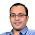Jan 28, 2010

Three NOT Gates from Two NOT Gates

Problem:

Design a 3-input 3-output logic circuit that negates the 3 signals. You have an infinite supply of AND and OR gates but only two NOT gates

I have read and solved many problems like these. Can people post some similar interesting problems using gates. I was asked one such question in my Deutsche Bank Interview which I was not able to answer.

Update(09/02/10):
Solution: Solution posted by Sid in comments!!

Update(23/06/14):

1.Here is 1 possible implementation

use of two NOTs to generate following fuction

(abc)'=a'+b'+c'=P
(ab+bc+ca)'=a'b'+b'c'+c'a'=Q

Now Pbc+Q=a'bc +a'b'+b'c'+c'a'=a'
Pac+Q=ab'c +a'b'+b'c'+c'a'=b'
Pab+Q=abc' +a'b'+b'c'+c'a'=c'

2.@piyush.. there is a mistake in your solution.

Pbc+Q = a'+b'c' and not a'
:(

3.@ Pratik : Deoes the three output terminals haeve to be specified as a',b' and c'
or can the output terminals produce these three bits in any order? In other words, is it necessary that always Terminal A produces a',B produces b' and C produces c' or just sufficient that A B and C have a' b' and c' at their outputs?

4.@Ankush..
Terminal A, B, C are just names. You can move "your wires" in whatever way u want. Given x_1, x_2, x_3.. I must receive x_1', x_2', x_3' :)

5.a,b,c are inputs:
define d = (ab+bc+ca)'
and e = (d.(a+b+c)+abc)'
then
a' = d(b+c+e) + ebc
b' = d(a+c+e) + eca
c' = d(a+b+e) + eab

6.@sid : How do you logically arrive at the solution??

7.d' is true iff at least 2 of a,b,c are true

d is false iff at least 2 of a,b,c are true

d is true iff at max 1 of a,b,c are true

d.(a+b+c) is true iff at least one of a,b,c are true and at max 1 of a,b,c is true

d.(a+b+c) is true iff exactly one of a,b,c is true

e' is true iff either 1 or all of a,b,c are true

e is true iff either 2 or none of a,b,c are true

Case1: 2 of a,b,c are true
a' is true if ebc is true

Case2: 1 of a,b,c are true
a' is true if d is true and b+c is true

Case3: None of a,b,c are true
a' is true if d is true and e is true

Case4: All of a,b,c are true
a' true is not possible

So, a' = d(b+c+e) + ebc
and alike

Awesome solution Sid.. Thanx..

Do you have similar problems? I am generally not able to solve such problems? Is there some strategy that people use? Thanx

8.9.There was a lot of trial and error, but the idea was this:
If we can make a',b' and c' then we can make all minterms. So try to make all minterms. Draw karnough map. Now initially you have a,b and c as inputs and operations allowed are AND/OR. Now we have to add two more inputs i.e d and e to this set of {a,b,c} to be able to make all the minterms. All minterms can be obtained by taking intersection of some of the inputs(useless to have a OR operation to get a minterm). From a,b,c we can only make one minterm i.e. abc. Now it is intuitive to assume that we should not use a minterm as d or e (we would be increasing the no of possible minterms produced by only 1). So a'b'c' has to be equal to d intersection e, as a'b'c' does not lie in either of a,b or c(in karnough map). Hence the intersection of d and e is exactly a'b'c. The rest follows

10.Nice explanation. Thanx

11.a'=(a+b+c)'+[(ab+bc+ca)'(b+c)]+bc
b'=(a+b+c)'+[(ab+bc+ca)'(c+a)]+ca
c'=(a+b+c)'+[(ab+bc+ca)'(a+b)]+ab

I'm afraid, Sid's solution work outs to give a'(b'+c'), b'(c'+a') & c'(a'+b') respectively...

I did the problem in this way:
1. Draw the K-Map for a', b' & c'...
2. Note that the column a'b'c' comes in common in all the 3 maps...
3. (a+b+c)'=a'b'c'{OR-ing the AND-ing of the 3 variables with 1 taken at a time & NOT-ing the result...}
4. (ab+bc+ca)'=a'b'+b'c'+c'a'{OR-ing the AND-ing of the 3 variables with 2 taken at a time & NOT-ing the result...}
5. R.H.S of 4, when AND-ed with a, b & c gives ab'c', a'bc' & a'b'c respectively...
6. Inspecting the K-Map for a', you can see that a' can be obtained by OR-ing a'bc', a'b'c, bc & our common term a'b'c'...
7. Using 3, 5 & 6, we get a' as given in the first line of this post...
8. Same is the case for b' & c'...

12.Solution using Prolog: http://quaxio.com/invert_three_signals/

1.I am not a prolog expert, but that looks detailed and elegant. Thank a ton

Fraction Brainteaser

Source: Sent to me by Gaurav Sinha Problem: Siddhant writes a Maths test and correctly answers 5 out of 6 Arithmetic questions and 20...